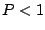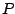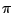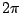Next: Applications Up: Designing filters Previous: Impulse responses of recirculating   Contents   Index

## All-pass filters

Sometimes a filter is applied to get a desired phase change, rather than to alter the amplitudes of the frequency components of a sound. To do this we would need a way to design a filter with a constant, unit frequency response but which changes the phase of an incoming sinusoid in a way that depends on its frequency. We have already seen in Chapter 7 that a delay of lengthintroduces a phase change of, at the angular frequency. Another class of filters, called all-pass filters, can make phase changes which are more interesting functions of.

To design an all-pass filter, we start with two facts: first, an elementary recirculating filter and an elementary non-recirculating one cancel each other out perfectly if they have the same gain coefficient. In other words, if a signal has been put through a one-zero filter, either real or complex, the effect can be reversed by sequentially applying a one-pole filter, and vice versa.

The second fact is that the elementary non-recirculating filter of the second form has the same frequency response as that of the first form; they differ only in phase response. So if we combine an elementary recirculating filter with an elementary non-recirculating one of the second form, the frequency responses cancel out (to a flat gain independent of frequency) but the phase response is not constant.

To find the transfer function, we choose the same complex numberas coefficient for both elementary filters and multiply their transfer functions:The coefficientcontrols both the location of the one pole (atitself) and the zero (at). Figure 8.23 shows the phase response of the all-pass filter for four real-valued choicesof the coefficient. At frequencies of,, and, the phase response is just that of a one-sample delay; but for frequencies in between, the phase response is bent upward or downward depending on the coefficient.Complex coefficients give similar phase response curves, but the frequencies at which they cross the diagonal line in the figure are shifted according to the argument of the coefficient.Next: Applications Up: Designing filters Previous: Impulse responses of recirculating   Contents   Index
Miller Puckette 2006-12-30